Search IntMath
Close

450+ Math Lessons written by Math Professors and Teachers

5 Million+ Students Helped Each Year

1200+ Articles Written by Math Educators and Enthusiasts

Simplifying and Teaching Math for Over 23 Years

# IntMath Newsletter: Quadratic graphs, Cymath, food and learning

By Murray Bourne, 30 Jun 2017

30 Jun 2017

1. New applet: Quadratic function graphs
2. Resource: Cymath
3. Math in the news: Math is not static
4. Math movie: Food and your brain
5. Math puzzles
6. Final thought: Age

First, two quick apologies:

• There's been a growing time gap between Newsletters. My consultancy work has been very busy lately and I haven't had as much time to devote to the Newsletter.
• The last edition, 11th May, was plagued by some technical issues and some of you received it twice, while others missed out. I did my best to rectify it.

## 1. New applet: What does b do in a quadratic function?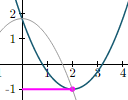The quadratic function y = ax2 + bx + c is a parabola. In this new applet, you can vary each of the parameters a, b and c using sliders. Changing the variables a and c are fairly simple to understand, but what does b actually change? See:

[Hat-tip to PiPo for the idea behind this applet.]

## 2. Resource: Cymath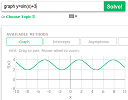Cymath is a free math solver, covering a broad range of topics including logarithms, trigonometry, calculus and of course, algebra. Similar to Wolfram|Alpha or the commercial Mathway solver, you enter your math question in the box and it provides an answer. But in Cymath's case, you get a full step-by-step solution for free.

Cymath is quick, and solutions for the most part appear to be mathematically sound. As they say on their About page:

Our math solver is powered by a combination of artificial intelligence and heuristics, so that it solves math problems step-by-step like a teacher would.

The site also provides a useful Reference section (containing common formulas with examples) and a Practice section (with common text book style questions, with solutions). There are Android and iOS mobile apps as well.

Cymath raises some interesting issues. Unfortunately, most students will use such a facility to complete their math homework for them (thus gaining marks, but not knowledge), but some will find the step-by-step solutions useful for their learning.

However, there are more issues involved here.

a. Why do math? The Cymath solver (like the other two mentioned) does quite a good job of giving the correct mathematical answer (the "what") and to some extent, the process for getting that answer (the "how"), but doesn't provide any indication of why we are doing the particular piece of mathematics or how to interpret the answer. (To be fair, I wouldn't expect them to do so - I'm just pointing out an issue.)

Going back to Cymath's own statement, if a math teacher just provides students with the algebraic steps for finding a solution, then they're doing a poor job of teaching that topic.

b. What math should we do? Since these tools are now readily available, why do we still make students spend most of their time doing the algebraic steps needed for the final answer? Surely it would be better to allow students to use the available algebra solvers, and spend most of their time learning how to solve actual application problems? For example, one of the example topics in Cymath is polynomial division. It doesn't really make sense to get students to learn how to find (x^3-1)/(x+2), when one of the main reasons for doing so is to solve polynomial equations. Such questions are usually very contrived (with "nice" numbers) and quite unrealistic anyway. We can ask the solver directly to solve such things using a statement like:

solve x^3+x^2-17x+13=0

It finds the 3 solutions numerically in an instant and frees us up to think about the implications of the answer.

## 3. Math in the news: Math is not static

In school mathematics, we often get the feeling that mathematics is some fixed, static entity that has not changed in hundreds of years. However, new math is being developed all the time. Here are two examples.

### a. New statistics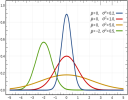A drug researcher usually assumes the response to a new drug will be the same for the experimental group as for the control group. But what if that is not the case? Also, how should we deal with outliers in our experimental results? Usually they are discarded, but is this the best approach?

In New statistical methods would let researchers deal with data in better, more robust ways Rand Wilcox, Professor of Statistics, University of Southern California points out that a number of new statistical techniques have been developed over the last 3 decades to better handle issues that arise when comparing groups of individuals or things, and the relationships between those groups.

The problem is, he says, researchers aren't using those new techniques becuase they don't know about then, and the techniques are not being taught in undergraduate statistics courses.

### b. A new 'branch' of mathEver notice the branches of a river look similar to the branches of a leaf, the roots of a plant or our blood vessels? They all tend to branch out at similar angles.

In A new 'branch' of math, we read that MIT researcher Dan Rothman used mathematical time modelling to examine how groundwater erodes surrounding hills, evnetually splitting a valley into 2 branches. Rothman's team determined there is a special angle (72°) at which streams branch. They confirmed this by examining 5,000 stream junctions in the Florida Panhandle.

Here's a related scientifc paper explaining how this process works in blood vessels: Quantification of Blood Flow and Topology in Developing Vascular Networks.

## 4. Math movie: How the food you eat affects your brainMany students have a terrible diet and it probably makes learning more difficult for them. In this short TED-Ed video, Mia Nacamulli explores the effects on our brains (and our learning abilities) depending on what we eat. Food for thought...

## 5. Math puzzles

The puzzle in the last IntMath Newsletter asked how many ancestors a male bee has.

Correct answers with explanation were provided by Isabel, Francis, and Jagmeet. This was a case of Fibonacci Series, of course.

### New math puzzle: Walking

A woman sets out on a "power walk" from her home and returns 6 hours later. If she walks 8 km/h on level land, 6 km/h uphill, and 12 km/hr downhill, how far did she walk?

You can leave your responses here.

## 6. Final thought: Age

Here's an age equation that's pretty accurate: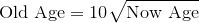When you're 16, old people are 40. When you're 50, old people are 70, and when you're 100, you know you’re old!

Until next time, enjoy whatever you learn.

See the 6 Comments below.

### 6 Comments on “IntMath Newsletter: Quadratic graphs, Cymath, food and learning”

1. Den Ducoff says:

Cymath looks good Murray, I would not have discovered this if not for your newsletter. Thanks again,

Den

2. Chris says:

Enjoyed this weeks problem 🙂
Distance eqn: 8l + 6u + 12d = t
Time eqn: l + u + d = 6
Trick is uphill distance equals downhill: 6u = 12d
Solve for t = 48 km

l -> hours walking on level
u -> hours walking uphill
d -> hours walking downhill
t -> total distance

3. Thomas A Buckley says:

The walked distance was 48 Km, because:-

For an out and back walk, let d Km = total up distance and also = total down distance.
As ups out equals downs on return, and vice versa. So total ups= total downs.
So total of up + down distances = 2d Km
and total time for up and down = d Km/6Km/h + d Km/12 Km/h = 0.25d hours.
Average speed for up and down = distance/ time = 2d Km/0.25d hours = 8Km/h
But level speed also =8Km/h.
So average speed for 6 hour walk = 8Km/h.
Therefore walk distance = Speed X Time = 8Km/h X 6 hours = 48 Km

4. Nash Shrestha says:

I got 48 km.

Let x be the distance she travelled on level ground, and y_up be the distance she travelled up the hill and y_down be the distance she travelled down the hill. Now, I assume that the hill is symmetric so that y_up = y_down = y (i.e. she travels the same distance up the hill and down the hill). We know that the whole trip from her home to the other side of the hill, and back again took 6 hours. So:

From her home to the other side of the hill: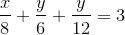From the other side of the hill back to her home: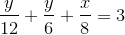Combining the two, we have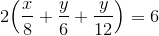or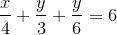Multiplying through by the LCM of 4,3, and 6 (which is 12), I got: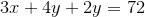and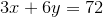so that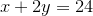. Now, we know that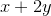is a one-way trip from her home to the other side of the hill. Then, the round trip would be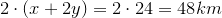So the distance she travelled is 48 km.

5. emmanuel kuria says:

looks like the variable b in the quadratic; changes the gradient of the straight line tangent to the parabola at the pole.

6. mkarimazmy says:

she walked 3 h and return in 3 h
8+6+12= 26 km is a simple answer

### Comment Preview

HTML: You can use simple tags like <b>, <a href="...">, etc.

To enter math, you can can either:

1. Use simple calculator-like input in the following format (surround your math in backticks, or qq on tablet or phone):
a^2 = sqrt(b^2 + c^2)
(See more on ASCIIMath syntax); or
2. Use simple LaTeX in the following format. Surround your math with $$ and $$.
$$\int g dx = \sqrt{\frac{a}{b}}$$
(This is standard simple LaTeX.)

NOTE: You can mix both types of math entry in your comment.